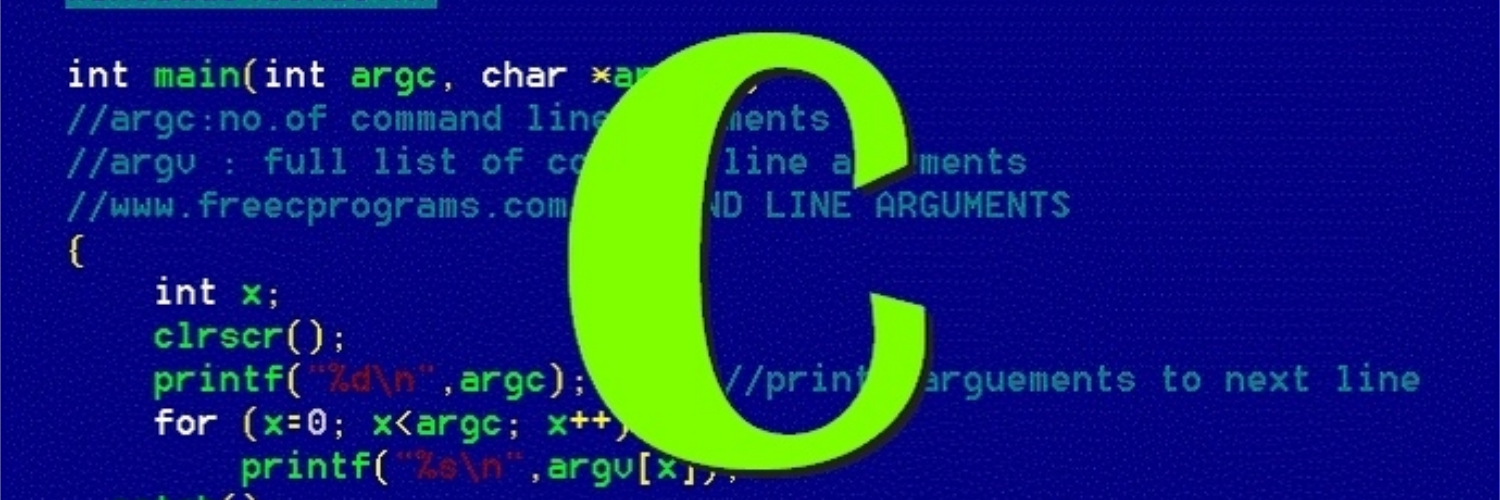# C Programming

## How Scanf Works

Shows in detail how scanf works and its gotchasscanf is essentially a “pattern matching” function that tries to match up groups of input characters with conversion specifications. An Example No blah blah blah, let’s see an example. int i, j; float x, y; scanf("%d%d%f%f", &i, &j, &x, &y); // input // <space><space>1-20.3-4.0e3<ret> Here is how scanf would process the new input: Skips the leading 2 spaces. Conversion specification: %d. The first nonblank input character is 1; since integers can begin with 1, scanf then reads the next character, -.

## C Programming - A Modern Approach

Book recommendation on learn C

I’m brushing up my C now using the book C Programming - A Modern Approach by K.N.King. I have the classic K&R book, I do not recommend it. I just find it very hard to read, no matter if you are a novice or a veteran. After some googling on people’s recommendations, I got the K.N.King book. I’m glad that I did. Very easy to read, has a good set of exercises and programming projects.

## Practical C - Stack

Implement Stack in CIn this blog post, let’s implement a generic stack in C. We will do this by several iterations, from simple version to the full blown version step by step. An Integer Version Let’s start with implementing an integer stack. #include <assert.h>#include <stdio.h>#include <stdlib.h> typedef struct { // int array to store elements of the stack int *elems; // the actual length of the stack int logicalLen; // the allocated length of the array int allocLen; } stack; // Initialize a stack void StackNew(stack *s); // Dispose a stack void StackDispose(stack *s); // Push an element onto the stack void StackPush(stack *s, int value); // Pop an element from the stack int StackPop(stack *s); void StackNew(stack *s) { // allocate 4 elements for the array s->elems = (int *)malloc(4 * sizeof(int)); s->allocLen = 4; s->logicalLen = 0; } void StackDispose(stack *s) { free(s->elems); } void StackPush(stack *s, int value) { if (s->logicalLen == s->allocLen) { // double the size of the array if there is no free space in it s->elems = (int *)realloc(s->elems, 2 * s->allocLen * sizeof(int)); s->allocLen *= 2; } s->elems[s->logicalLen] = value; s->logicalLen++; } int StackPop(stack *s) { assert(s->logicalLen !

## Practical C - Linear Search

Implement linear search in CI start this blog series to show some of the trickier parts of C programming. In the first post, let’s implement the linear search in C. Integer Version Let’s imagine that we search on an integer array. Two things to notice here: The function needs the array size n as a separate parameter. Only the array’s address is passed in the function. Generic Basic Version Let’s write a more generic version that does not specify type.

## Generic Programming in C

How to do generic programming in C